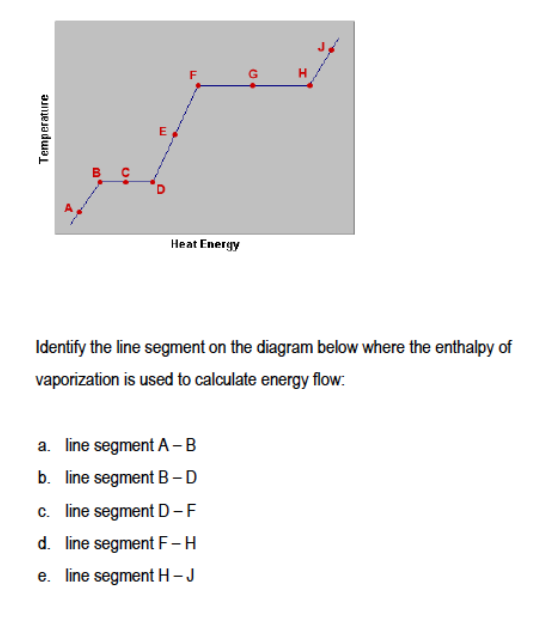# Problem: Refer to the diagram below for the following questions:

###### FREE Expert Solution
86% (469 ratings)
###### Problem Details

Refer to the diagram below for the following questions:Frequently Asked Questions

What scientific concept do you need to know in order to solve this problem?

Our tutors have indicated that to solve this problem you will need to apply the Heating and Cooling Curves concept. You can view video lessons to learn Heating and Cooling Curves. Or if you need more Heating and Cooling Curves practice, you can also practice Heating and Cooling Curves practice problems.

What is the difficulty of this problem?

Our tutors rated the difficulty ofRefer to the diagram below for the following questions:   ...as medium difficulty.

How long does this problem take to solve?

Our expert Chemistry tutor, Jules took 1 minute and 31 seconds to solve this problem. You can follow their steps in the video explanation above.